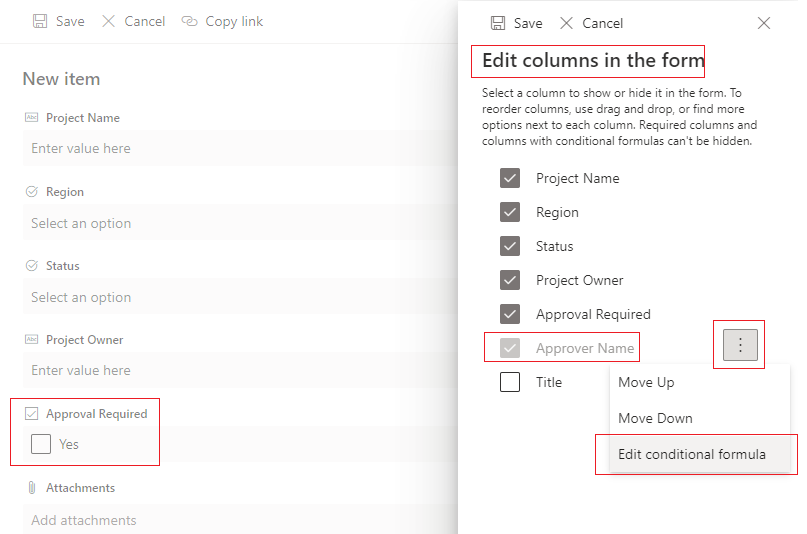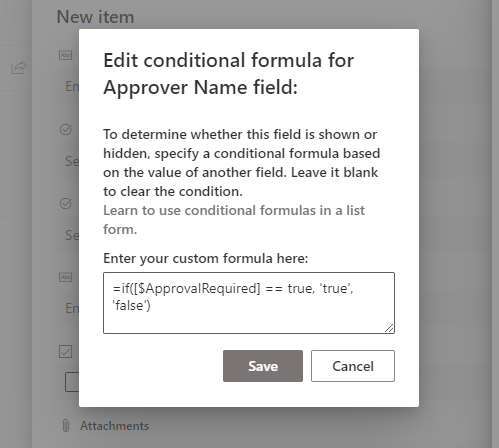# Show Hide SharePoint column in List Library form with the conditional formula

SharePoint Modern view provides an OOTB (Out Of The Box) way to show or hide columns in a List or Document Library form based on value for another field. You can use a formula to perform condition based checks on values in a SharePoint column.

### ✔️ Steps to show or hide columns on SharePoint from based on condition

1. Navigate to your respective list or library.
2. Click 'New' or open any List Item (or document properties) to view the Display form.
3. Click "Edit form" from the top right corner of the form.
4. Click "Edit Columns".
5. Click on menu option (...) which is at the far right side of the column name.
6. In the dialog popup, enter the custom condition formula, which will show/hide this current column based on the value of another column.
7. Click Save.

### ⚡️ Example

Assume we have a SharePoint List for maintaining project details. The form asks user if 'approval is required ?' (Yes/No field) for the project. If approval required is Yes, then 'Approver Name' field is shown; if No, then the Approver field is hidden.SharePoint Online column conditional formula

Use the earlier steps to add conditional formula for the 'Approver Name' column (as this is the field for which we want to toggle the visibility), copy and paste below formula. Change the field name if you are using a different name.
``=if([\$ApprovalRequired] == true, 'true', 'false')``SharePoint conditional formula to show hide column

### ⭐ Points to consider

• Conditional show/hide of columns works on New and Edit forms, not on display because its only a view form.
• The conditional formula does not work with Quick Edit view (Edit in grid view).
• Comparison and evaluation values true and false are case-sensitive, type in lower case.
• The condition formula works only when it returns true or false values.
• Refer to the field with internal name in this format - [\$FieldInternalName].
• This approach hides the respective field in the New/Edit forms and not from the view. To hide the field from the view, modify the view.
• These column types are not supported
• Person or Group with multiple selections
• Choice with multiple selections
• Time calculations in Date and Time column
• Currency columns
• Location columns
• Calculated columns

### ✌️ Conditional formulas examples

Single line of text / Choice - equals
``=if([\$ProjectCategory] == 'Information Technology', 'true', 'false')``

Number - greater, less, equal
``=if([\$ProjectCost] >= 5000, 'true', 'false')``

Number - sum
``=if(([\$Cost] + [\$Tax]) > 1000, 'true', 'false')``

Yes/No - Yes or No (boolean)
``=if([\$ApprovalRequired] == true, 'true', 'false')``

Person - email account equals
``=if([\$Approver.email] == 'c2cAdmin@code2care.com', 'true', 'false')``

Date - equal, less, greater (check the date format)
``=if([\$ProjectKickoffDate] == Date('3/7/2021'), 'true', 'false')``

Date - between 2 dates
``=if([\$ProjectKickoffDate] >= Date('6/10/2021') && [\$ProjectKickoffDate] <= Date('7/10/2021'), 'true', 'false')``

Lookup - equals (check the lookup value)
``=if(substring([\$Category],indexOf([\$Category],';#')+2,500) == 'Migration', 'true', 'false')``

Lookup - equals (check the lookup id)
``=if(Number(substring([\$Category],0,indexOf([\$Category],';#'))) == 1, 'true', 'false')``

Have Questions? Post them here!